# CBSE Solutions for Class 10 English

#### GSEB std 10 science solution for Gujarati check Subject Chapters Wise::

The SI unit of resistivity is ……… .

જવાબ : ohm-metre (Ω m)

The resistance of a conductor depends directly on its ……… , inversely on its ……… and also on the ……… of the conductor.

જવાબ : length, area of cross-section, material

According to ……… Law, the potential difference across the ends of a resistor is directly proportional to the ……… through it, provided its remains constant.

જવાબ : Ohm’s, current, temperature

The SI unit of current is ……… .

જવાબ : ampere

Presence of chemically inactive gases like argon and …………… prolong the life of the filament.

જવાબ : Nitrogen

The rate at which electric energy is consumed or dissipated is called ……………

જવાબ : Electric power

Copper and aluminium are used for electric transmission lines because both posses …………… resistivity.

જવાબ : Low

Ammeter is used to measure the magnitude of …………… through any wire of a circuit.

જવાબ : Electric Current

…………… measures the potential differences between two points of the circuit.

જવાબ : Voltmeter

Electric current flows in the circuit from …………… terminal of cell to the …………… terminal of cell.

જવાબ : Positive, Negative

Write down the relation between the potential difference between two points A and B in a conductor, work done W in moving a unit charge from point B to A and the charge q.
Or
State the relation between work, charge and potential difference for an electric circuit.
[CBSE (All India) 2009, 2013]
Or
Express work done in an electric field in terms of charge and potential difference. (CBSE 2014)

જવાબ :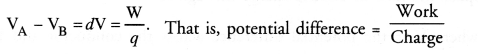Name the instrument used to measure the electric potential difference. (CBSE 2011, 2012)

જવાબ : Voltmeter.

What is meant by potential difference between two points ? (CBSE 2011)

જવાબ : Work done per unit charge in moving a unit positive charge from one point to another point in an electric field is called potential difference between two points.

Is electric potential a scalar or a vector physical quantity ?

જવાબ : Electric potential is a scalar physical quantity.

Define 1 volt electric potential.

જવાબ : Electric potential is said to be 1 volt if 1 Joule of work is done in moving 1 coulomb charge from infinity to a point in the electric field.

What is the S.I. unit of electrical potential ? (CBSE 2005, 2012)

જવાબ : volt.

State the relation between work (W), change (q) and electric potential (V).

જવાબ : V = W/q.

Define electric potential.

જવાબ : Electric potential at a point in an electric field is defined as the work done in moving a unit positive charge from infinity to that point in the electric field.

If the potential difference across the ends of a conductor is doubled, the current flowing through it, gets ……… .

જવાબ : doubled

Mention the factor that maintains the flow of charge through a conductor. (CBSE 2013, 2014)

જવાબ : Potential difference across the ends of the conductor.

Define electric current.

જવાબ : Electric current is defined as the amount of electric charge flowing through any cross-section of a conductor per unit time.

Write down the relation between the electric current I passing in a conductor, change Q flowing in the conductor and time t.
Or
Write the relation between coulomb and ampere. (CBSE 2014)

જવાબ :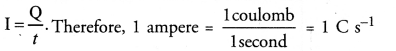Define 1 ampere electric current.

જવાબ : Electric current through a conductor is said to be 1 ampere if 1 coulomb electric charge flows through a cross-section of a conductor in 1 second.

State SI unit of electric current.

જવાબ : ampere (A)

State the relation between the current I passing in a conductor, number of electrons (n) flowing through any cross-section of the conductor, magnitude of charge on an electron (e) and time t.

જવાબ : I = ne/t.

Define ohm’s law. [CBSE 2010, 2011, 2012, 2013, 2015]
Or
State the law that gives the relationship between the potential difference (V) across the two ends of a conductor and the current (I) flowing through it. (CBSE 2012)

જવાબ : Ohm’s law states that the electric current flowing through a conductor is directly proportional to the potential difference across the ends of the conductor, provided the temperature and . other physical conditions of the conductor remain the same.

How is voltmeter connected in the circuit to measure electric potential difference ?

જવાબ : Voltmeter is connected in parallel across a conductor or resistor in the electric circuit.

How is ammeter connected in the circuit to measure electric current ? (CBSE 2011, 2012)

જવાબ : Ammeter is connected in series in an electric circuit.

Name the instrument used to measure electric current in a circuit. [CBSE (Delhi) 2008, 2011, 2012, 2015]

જવાબ : Ammeter.

How is the direction of electric current related to the direction of flow of electrons in a wire ? [CBSE (All India) 2009]

જવાબ : The direction of electric current in a wire is just opposite to the direction of flow of electrons in the wire.

Graph is plotted between the values of potential difference (V) and current (I). What v conclusion do you draw about the relation between V and I from this graph. State this relation in your words. (CBSE 2013)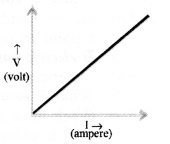જવાબ : The potential difference (V) is directly proportional to the current (I).

Draw a circuit diagram to establish a relationship between potential difference across the ends of a resistor and current flowing through it. (CBSE 2013)

જવાબ :State the formula showing how the current I in a conductor varies with the potential difference V applied across it. [CBSE (Delhi) 2004(S), 2012]

જવાબ : V I or V = IR or I = V/R .

Define electrical resistance of a conductor. (CBSE 2013)

જવાબ : It is the property of a conductor to oppose the flow of electric charge through it.
Resistance of a conductor, R = V/I , where V is the potential difference across the conductor and I is the current flowing through the conductor.

State SI unit of resistance. [CBSE (Delhi) 2004(S), 2012]

જવાબ : ohm (Ω).

What does the slope of V-I graph at any point represent ?

જવાબ :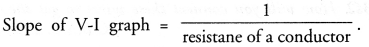Therefore, slope of V-I graph represents reciprocal of the resistance of a conductor resistance of a conductor.

What is the name the physical quantity which is equal to V/I ?
Or
Name the physical quantity whose unit is volt-ampere.

જવાબ : y = R. Therefore, physical quantity is resistance of a conductor.

What is the shape of the graph obtained by plotting potential difference applied across a conductor against the current flowing through it ?

જવાબ : A straight line passing through the origin and has constant slope.

“The resistance of a conductor is IΩ”. What is meant by this statement ? (CBSE 2010, 2011, 2012)
Or
Define 1 ohm resistance. (CBSE 2014)

જવાબ : The resistance of a conductor is said to be 1Ω if a potential difference of IV across the ends of the conductor makes a current of 1A to flow through it.

The voltage — current (V-I) graph of a metallic circuit at two different
temperature T1 and T2 is shown. Which of the two temperatures is higher and why ? (CBSE 2010)
Or
The voltage-current (V-I) graph of a metallic conductor at two different temperatures T1 and T2 is shown in figure. At which temperature is the resistance higher ? (CBSE 2011, 2012)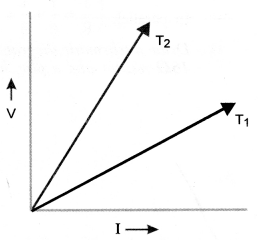જવાબ : Slope of I-V graph = resistance of metallic conductor.
Since, slope of I-V graph at temperature T2 is greater than the slope of I-V graph at temperature T1, therefore, resistance at T2 is greater than resistance at T1 . Since, resistance of a metallic conductor increases with increase in temperature, therefore, T2 > T1.

What happens to resistance of a conductor when temperature is increased ?

જવાબ : Resistance of a conductor increases with the increase in temperature.

State the relation between the resistance R of a conductor, resistivity ρ of a conductor, length l of a conductor and area of cross-section A of the conductor.
Or
Write an expression for the resistivity of a substance. (CBSE 2015)

જવાબ :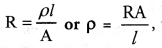where R is the resistance, A is the area of cross-section and l is the length of the substance.

How does the resistance of a wire vary with its cross-section area ?

જવાબ : Resistance of a wire is inversely proportional to its cross-sectional area. More is cross-sectional area of a conductor, less is the resistance of the conductor.

n resistance each of R are connected in series in a circuit. What is the total effective resistance of the circuit ?
(CBSE 2010)

જવાબ : Reff = R1 + R2 + R3 + … upto n = nR

You have a metal, insulator and an alloy. Write these substances in the ascending order of their electrical resistivity.

જવાબ : Resistivity of metal < resistivity of alloy < resistivity of insulator.

A wire of resistivity ‘ρ’ is pulled to double its length. What will be its new resistivity ? (CBSE 2010, 2011)

જવાબ : New resistivity will also be ‘ρ’ because resistivity of a wire does not depend on its length.

State SI unit of resistivity. [CBSE (Delhi) 2004 (S), 2014, 2015]

જવાબ : ohm-metre (Ω-m).

What is electrical resistivity ? [CBSE 2010, 2014, 2015]

જવાબ : Electrical resistivity of a material is defined as the resistance of an object (made of the material) of unit length and unit area of cross-section.

n resistors each of resistance R are connected in parallel in an electric circuit. What is the total effective resistance of the circuit ? (CBSE 2010 )

જવાબ :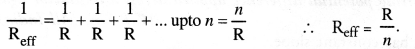n resistors each of resistance R are first connected in series and then in parallel. What is the ratio of the total effective resistance of the circuit in series combination and parallel combination ? (CBSE 2013)

જવાબ :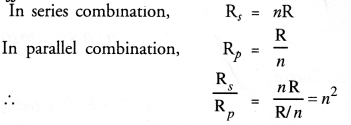In the given circuit, connect a nichrome wire of length ‘L’ between points X and Y and note the ammeter reading.
(i) When this experiment is repeated by inserting another nichrome wire of the same thickness but twice the length (2L), what changes are observed in the ammeter reading?
(ii) State the changes that are observed in the ammeter reading if we double the area of cross-section without changing the length in the above experiment. Justify your answer in both the cases.
(b) “Potential difference between points A and B in an electric field is 1 V”. Explain the above statement.જવાબ : (a)(i) Ammeter reading in the second case is half of the ammeter reading
in first case. This is because
=> i.e. when length is doubled, the resistance is doubled, this means the current is halved.
(ii) On doubling the area of cross-section without changing the length of the conductor, twice of the previous reading is observed in the ammeter. This is because
So, when A is double, resistance becomes half, current will be doubled. (b) “Potential difference between points A and B in an electric field is 1 V”. It means 1 J work is done in moving 1 C of charge from point B to point A in an electric field.

(a) Though same current flows through the electric line wires and the filament of bulb, yet only the filament glows. Why?
(b) The temperature of the filament of bulb is 2700°C when it glows. Why does it not get burnt up at such high temperature?
(c) The filament of an electric lamp, which draws a current of 0.25 A is
used for four hours. Calculate the amount of charge flowing through the
circuit.
(d) An electric iron is rated 2 kW at 220 V. Calculate the capacity of the fuse
that should be used for the electric iron.

જવાબ : (a) Electric line wires offer extremely low resistance to the flow of current, so
they do not glow because negligible heat is produced in it.
The filament of bulb glows because it becomes red hot due to large amount of
heat produced, as it offers high resistance to the flow of current through it.
(b) The filament of bulb when it glows at 2700°C does not get burnt because the tungsten metal of filament has
(i) a very high melting point (of 3380°C) and
(ii) a high resistivity.(c) Given: I = 0.25 A, t = 4 h = 4 x 60 x 60 sec.
So, amount of charge flowing the filament of electric lamp
So, the capacity of the fuse that should be used for the electric iron is of the order of 10 A.

Two identical wires one of nichrome and other of copper are connected in series and a current (I) is passed through them. State the change observed in the temperatures of the two wires. Justify your answer. State the law which explains the above observation.

જવાબ : The resistivity of nichrome is more than that of copper so its resistance is also high. Therefore, large amount of heat is produced in the nichrome wire for the same current as compared to that of copper wire. Accordingly, more change in temperature is observed in the nichrome wire. This is explained by Joule’s law of heating.

Joule’s law of heating: It states that the amount of heat produced in a conductor isAn electric bulb is rated at 60 W, 240 V. Calculate its resistance. If the voltage drops to 192 V, calculate the power consumed and the current drawn by the bulb. (Assume that the resistance of the bulb remain unchanged.)

જવાબ :Three bulbs each having power P are connected in series in an electric circuit. In another circuit, another set of three bulbs of same power are connected in parallel to the same source.
(i) Will the bulbs in both the circuits glow with the same brightness? Justify your answer.
(ii) Now let one bulb in each circuit get fused. Will the rest of the bulbs continue to glow in each circuit? Give reason.
(iii) Representing each bulb by a resistor, draw circuit diagram for each case.

જવાબ : (i) Bulbs in parallel provide more illumination. This is because
(a) each bulb gets same voltage and is equal to the applied voltage.
(b) each bulb draws required current from the mains. Hence, they work properly.
(ii)When one bulb in each circuit get fused,
In series: Rest of the bulbs will not glow. This is because in series arrangement, there is only a single path for the flow of current.
In parallel: Rest of the bulbs will continue to glow as in parallel connection,
(a) individual branch in the circuit completes its own circuit, or
(b) different paths are available for the flow of current.
(iii) Circuit diagramAn electric bulb of resistance 200Ω draws a current of 1 Ampere. Calculate the power of the bulb the potential difference at its ends and the energy in kWh consumed burning it for 5h.

જવાબ : Power of the bulb,(a) Define electric power. Express it in terms of potential difference V and resistance R.
(b) An electrical fuse is rated at 2 A. What is meant by this statement?
(c) An electric iron of 1 kW is operated at 220 V. Find which of the following fuses that respectively rated at 1 A,3 A and 5 A can be used in it.

જવાબ : (a) Electric power: It is the rate of doing work by an energy source or the rate at which the electrical energy is dissipated or consumed per unit time in the electric circuit is called electric power.Two wires A and B are of equal length and have equal resistance. If the resistivity of A is more than that of B which wire is thicker and why? For the electric circuit given below calculate:(i) Current in each resistor,
(ii) Total current drawn from the battery, and
(iii) Equivalent resistance of the Circuit

જવાબ :So, for different materials having same resistance per unit length, greater resistivity material wire has more cross-sectional area.
Hence, wire A is thicker than that of B.
(i) Current through each resistorDraw a labelled circuit diagram showing three resistors R1, R2 and R3 connected in series with a battery (E), a rheostat (Rh), a plug key (K) and an ammeter (A) using standard circuit symbol. Use this circuit to show that the same current flows through every part of the circuit. List two precautions you would observe while performing the experiment.

જવાબ :Aim: Same current flows through every part of the above circuit.
Procedure:

1. Connect ammeters, ‘A’1 between B and C, and ‘A2’ between D and E.
2.  Adjust the sliding contact of the rheostat initially for a small current.
3. Note all the ammeter readings. These reading give us current flowing through the resistors R1, R2 and R3
4. The current in the circuit is now increased by changing the position of sliding contact J’ of the rheostat.
5. Note all the ammeter readings each time.
Conclusion: Same reading of all the ammeter in each observation concluded that same current flows through every part of the circuit.

Precautions:

1. All the connection should be tight and properly connected as per circuit diagram.
2. The positive terminal of the ammeter and voltmeter must be connected to the positive terminal of the battery or battery eliminator.

V-I graph for two wires A and B are shown in the figure. If both wires are of same length and same thickness, which of the two is made of a material of high resistivity? Give justification for your answer.જવાબ : Greater than slope of V-I graph, greater will be the resistance of given metallic wire. In the given graph, wire A has greater slope then B. Hence, wire A has greater resistance.
For the wires of same length and same thickness, resistance depends on the nature of material of the wire, i.e.State Ohm’s law. Write the necessary conditions for its validity. How is this law verified experimentally? What will be the nature of graph between potential difference and current for a conductor? Name the physical quantity that can be obtained from this graph.

જવાબ : Ohm’s law : When the physical conditions such as temperature etc. remain same, the current flowing through the conductor is directly proportional to the potential difference applied across the ends of the conductor, i.e.,Necessary condition for validity of Ohm’s law is that physical condition such as
temperature of the conductor remains same.
Procedure:
(i) Complete the circuit by connecting one cell in the gap XY. Plug the key.(ii) Note the reading in the ammeter for the current I and in the voltmeter for the potential difference, (V) across the nichrome wire.(v) Find the ratio of F to / for each observation.
(vi) Plot a graph between V (y-axis) and I (x-axis).
Observation :

1. Voltmeter and ammeter reading increases as the number > of cells increase in series.
2. Same value of V/I is obtained in each case.
3.  V-I graph is a straight line passing through the origin of the graph as shown.Conclusion : Straight line nature of graph shows that the current is proportional to the potential difference. Hence, Ohm’s law verified. The slope of V-I graph gives the value of resistance of the conductor at the given temperature.

What is meant by electrical resistivity of a material? Derive its S.I. unit. Describe an experiment to study the factor on which the resistance of a conducting wire depends.

જવાબ : Mathematically, resistivity of the conducting material is given by
p = R x A/J
If l = 1 m, A = 1 m2, then p = R
Hence, the resistivity of the material is defined as the resistance offered by a metallic wire having a unit length and a unit area of cross-section. Since unit length and unit area of cross-section forms a cube, the specific resistance or resistivity can also be defined as the resistance offered by a cube of a material of side 1 m when current flows perpendicularly through the opposite faces. In SI system, its units isExperiment:
Aim : To study the factors on which resistance of conducting wires depends. Apparatus Required : A cell, an ammeter, nichrome wires of different length but same area of cross-section (thickness), nichrome wires of same length but different thickness, copper and iron wire of the same length and same thickness as that of any nichrome wire.
Procedure :

1. Connect the cell, an ammeter and plug key in series with nichrome wire of length T (marked 1) in the gap XY as shown.2. Close the key and note the reading of ammeter. It measures the current ‘I’through the nichrome wire (marked ‘1’).
3. Replace the marked 1 wire with another nichrome wire having same area of cross-section (thickness) but of double length’2l’ (marked 2).
4. Note the ammeter reading (I2) again after closing the key.
5. Again replace the marked 2 wire with marked 3 wire which has the same length but is thicker than marked 1 and 2 nichrome wires. Again note down the current (I3) through this wire.
6. Unplug the key. Remove marked 3 nichrome wire from the gap XY. Connect the copper wire marked 4 having same length and same area of cross-section as that of nichrome wire marked 1. .
7. Plug the key again and note the ammeter reading. It measures the current (I4) through copper wire.
8. Repeat the experiment with iron wire and measure the current (I5).
Observation :

1. Current ‘ I’ is half ofI1 i.e., I2 =1/2 I1
2. Current I3 increases when thicker wire of same length and of same material
i. e., nichrome is used.
3. Current I4 and I5 is different for copper and iron wire.
Conclusion :

1. Different wires drew different amount of current from the same cell.
2. First observation indicates that the resistance of the conductor increases with increase in length. So, resistance is directly proportional to length.
3. Second observation shows that thicker wires have lesser resistance. So, resistance is inversely proportional to area of cross section of the wire.
4. Third observation shows that resistance of the conductor depends on the nature of its material.

What is meant by electric current? Name and define its SI unit. In a conductor electrons are flowing from B to A. What is the direction of conventional current? Give justification for your answer.
A steady current of 1 ampere flows through a conductor. Calculate the number of electrons that flows through any section of the conductor in 1 second. (Charge on electron 1.6 X 10-19 coulomb).

જવાબ :

• Electric Current: The amount of charge ‘Q’ flowing through a particular area of cross section in unit time ‘t’ is called electric current, i.e.
• Electric current, I = Q/t
•  SI unit of electric current is ampere.
•  One ampere of current is that current which flow when one coulomb of electric charge flowing through a particular area of cross-section of the conductor in one second, i.e. 1A = 1 Cs-1.
The direction of conventional current is A to B, i.e. opposite to the direction of flow of electrons. In a metal, flow of electrons carrying negative charge constitutes the current. Direction of flow of electrons gives the direction of electronic current by convention, the direction of flow of positive charge is taken as the direction of conventional current.
Charge = q = neFind the current drawn from the battery by the network of four resistors Shown in the figure.જવાબ :Study the following electric circuit and find (i) the current flowing in the circuit and (ii) the potential difference across 10 Ω resistor.જવાબ :(i) Draw a closed circuit diagram consisting of a 0.5 m long nichrome wire XY, an ammeter, a voltmeter, four cells of 1.5 V each and a plug key.
(ii)Following graph was plotted between V and I values :What would be the values of V /I ratios when the potential difference is 0.8 V, 1.2 V and 1.6 V respectively? What conclusion do you draw from these values?

જવાબ :State Ohm’s law. How can this law be verified experimentally ? Does Ohm’s law hold good under all conditions.
Comment. (NCERT Question Bank, CBSE, 2010, 2012)

જવાબ : For Ohm’s law: Ohm’s law states that the electric current flowing through a conductor is directly proportional to the potential difference across the ends of the conductor, provided the temperature and . other physical conditions of the conductor remain the same.
For experimental verification: Verify Ohm’s law
Apparatus : A conductor of resistance R, an ammeter, a voltmeter, a battery, a variable resistance (or rheostat used to change the current in the circuit), connecting wires, a key and sand paper.
Procedure:

1. Connect the various components as shown in figure 12.2. Close the key, so that current begins to flow in the circuit.
3. Note down the potential difference (V) across the conductor PQ of resistance R shown by the voltmeter and the corresponding current (I) shown by the ammeter.
4. Now move the knob of rheostat so that the current in the circuit increases.
5. Again note down the potential difference (V) across the conductor PQ of resistance R in the voltmeter and current in the circuit shown by ammeter.
6. Repeat the experiment at least five times by increasing the current in the circuit by moving the knob of the rheostat in steps.

Define electric power. A device of resistance R is connected across a source ofV voltage and draws a current I. Derive an expression for power in terms of voltage and resistance.

જવાબ : Electric power is defined as the amount of electric work or electric energy per unit time.
If W be the amount of electric energy consumed in a circuit in t seconds, then the electric power is given byTwo identical immersion heaters are to be used to heat water in a large container. Which one of the following arrangement would heat the water faster,

1. connecting the heaters in series with the main supply,
2. connecting the heaters in parallel with the main supply ? (CBSE 2011)

જવાબ : Heat produced in a heater, when connected to main supply,When identical heaters (i.e. having same resistance) are connected in parallel, their net resistance decreases as compared to when connected in series. Therefore, heaters connected in parallel would heat the water faster as the heat produced in parallel combination is more than the heat produced in series combination, on electric energy and electric power

Should the heating element of an electric iron be made of iron, silver or nichrome wire 1
[CBSE (Foreign)2005, 2016]
Or
List two reasons why nichrome is used for making heating element of electrical appliances. (CBSE 2015)

જવાબ : It should be made of nichrome wire because

1. resistivity of nichrome is greater than that of iron and silver,so more heat is produced in the nichrome wire due to the flow of current.
2. melting point of nichrome wire is greater than that of iron and silver and hence it does not melt easily on heating.
3. nichrome wire does not oxidise (or burn) easily even at higher temperature.

Draw diagrams to show series and parallel combinations of resistors. State three salient features each of both the combinations. (CBSE 2015)

જવાબ :Salient features of series combination :

1. The net resistance of the combination is equal to the sum of the resistances of the individual resistor.
2. The current flowing through each resistor is the same.
3. The voltage applied across the series combination of resistors is equal to the sum of potential differences across the individual resistors.
Salient features of parallel combination :

1. The reciprocal of the net resistance of the combination is equal to the sum of the reciprocal of the resistances of the individual resistors.
2. Different amount of current flows through each resistor.
3. The potential difference across each resistor is equal to the voltage applied across the combination.

Is the circuit given below correct ? Justify your answer. (CBSE 2014)જવાબ : The given circuit is incorrect because in a circuit, ammeter is always connected in series and voltmeter is connected parallel to the resistor R1. The correct circuit is shown in figure.List two distinguishing features between the resistance and resistivity of a conductor. (CBSE 2014)

જવાબ :

1. Resistance of a conductor depends on the length and area of cross-section of the conductor. On the other hand, resistivity of a conductor is independent of length and area of cross-section of the conductor.
Unit of resistance is ohm. Unit of resistivity is ohm-metre.

Two students perform experiments on two given resistors R1 and R2 and plot the following V-I graphs. If R1 > R2, which of the two diagrams correctly represent the situation on the plotted curves ? Justify your answer. (CBSE 2010, 2012)જવાબ : Resistance of a conductor = slope of I-V graph. It means, resistance is high if slope of I-V graph for it is steeper. Since R1 > R2, therefore, slope of I-V graph for R1 must be steeper than the slope of I-V graph for R2 Thus, diagram I represents the situation correctly.

In the circuit diagram shown, the two resistance wires A and B are of same area of cross-section and same material, but A is longer than B. Which ammeter A1 and A2 will indicate higher reading of current ? Give reason. (CBSE 2010, 2011)જવાબ : The current in the circuit is high if the resistance of the circuit is low. Since wire A is longer than B, so the resistance of wire B is less than the resistance of wire A. Hence, current flowing through wire B is greater than the current flowing through wire A. Therefore, ammeter A2 will indicate higher reading of current.

In the circuit diagram shown, the two resistance wires A and B are of the same length and same material, but A is thicker than B. Which ammeter Af or A2 will indicate higher reading for current ? Give reason.
(CBSE 2010, 2011)p and l for both wires A and B are same but area of cross-section (A) of wire A is- more than the wire B. Therefore, resistance of wire A is less than the resistance of the wire B. Hence, ammeter A connected in series with the wire A will indicate higher reading for current.

What do the following symbols represent in a circuit ? Write the name and one function of each ?
[CBSE 2011, 2012, 2013, 2015]જવાબ : (i) It represents a closed plug key. It is used to make the closed electric circuit.
(ii) It represents a variable resistance. It is used to increase or decrease the electric current in the circuit.
(iii) It represents a voltmeter. It is used to measure the potential difference across a resistor in the circuit.
(iv) It represents a galvanometer. It is used to detect the presence of small current in the circuit.
(v) It represents wire crossing (not connected with each other). The wires are used to connect various components in the circuit.

What is an electric circuit ? Distinguish between an open and a closed circuit.
[CBSE (All India) 2009, 2010, 2011]

જવાબ : electric circuit:
An electric circuit is a closed conducting path containing a source of electric energy (i.e., a cell or a battery) and a device or element or load (say, an electric bulb) utilizing the electric energy.
The direction of electric current is opposite to the direction of the flow of electrons in the conductor.
Open electric circuit : An electric circuit through which no electric current flows is known as open electric circuit.
The electric circuit shown in figure 10 (A) will be open circuit if the plug of the key is taken out or if the connecting wire breaks from any point.Closed circuit : An electric circuit through which electric current flows continuously is known as closed circuit (Figure 10 (B)).

List two differences between a voltmeter and ammeter. (CBSE 2013)

જવાબ :

 Ammeter Voltmeter 1.    Ammeter measures electric current in the circuit. 2.     Ammeter is connected in series in an electric circuit. 1.     Voltmeter measures the potential difference be­tween two points on a conductor. 2.     Voltmeter is connected in parallel across the ends of a conductor or resistor.

Define electric current. State and define its SI unit. (CBSE 2012, 2013, 2014, 2015)

જવાબ : Electric current is defined as the amount of charge flowing through a cross-section of a conductor in unit time.SI unit of electric current is ampere (A).
Electric current through a conductor is said to be 1 ampere if one coulomb charge flows through a cross-section of the conductor in one second.

### There are No Content Availble For this Chapter

Match the following columns

 Column I Column II (a) Resistance (i) Infinite (b) The rate of flow of charge (ii) Potential difference (c) The work done to move a unit charge (iii) Obstruction to the flow of current from one point to another (d) Resistance of ideal ammeter (iv) Watt (e) Resistance of ideal voltmeter (v) Electric current (f) SI unit of electric power (vi) Zero

જવાબ :

 Column I Column II (a) Resistance (iii) Obstruction to the flow of current from one point to another (b) The rate of flow of charge (v) Electric current (c) The work done to move a unit charge (ii) Potential difference (d) Resistance of ideal ammeter (vi) Zero (e) Resistance of ideal voltmeter (i) Infinite (f) SI unit of electric power (iv) Watt

 a. Bulb is usually filled with ampere b.  SI unit of current potential difference c.  Flow of current in a wire is due to nitrogen

જવાબ :

a. Bulb is usually filled with (nitrogen)
b. SI unit of current (ampere)
c. Flow of current in a wire is due to (potential difference)

 a. The device which measures current fuse b. The device which measures potential difference parallel c. The device which protect electric appliances from high current ammeter d. SI unit of voltage volt e. Combination used for household use kilowatt hour f . Commercial unit of energy voltmeter

જવાબ :

a. The device which measures current (ammeter)
b. The device which measures potential difference (voltmeter)
c. The device which protect electric appliances from high current (fuse)
d. SI unit of voltage (volt)
e. Combination used for household use (parallel)
f. Commercial unit of energy (kilowatt hour)